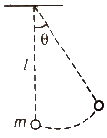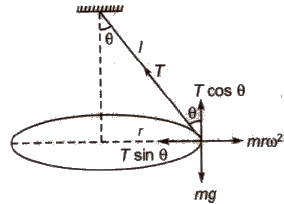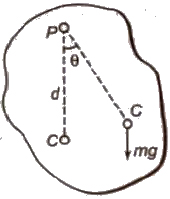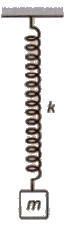Courses

# Types of Pendulums Class 11 Notes | EduRev

## JEE : Types of Pendulums Class 11 Notes | EduRev

The document Types of Pendulums Class 11 Notes | EduRev is a part of the JEE Course Physics Class 11.
All you need of JEE at this link: JEE

Simple Pendulum

A simple pendulum consists of a heavy point mass suspended from a rigid support by means of an elastic inextensible string.

The time period of the simple pendulum is given by :

T = 2π √l / gwhere l = effective length of the pendulum and g = acceleration due to gravity.

If the effective length l of simple pendulum is very large and comparable with the radius of earth (R), then its time period is given by

T = 2π √Rl / (l + R)g

For a simple pendulum of infinite length (l >> R)

T = 2π √R / g = 84.6 min

For a simple pendulum of length equal to radius of earth,

T = 2π √R / g = 60 min

If the bob of the simple pendulum is suspended by a metallic wire of length l, having coefficient of linear expansion α, then due to increase in temperature by dθ, then

Effective length l’ = l (1 + α dθ)

Percentage increase in time period

(T’ / T – 1) * 100 = 50 α dθ

When a bob of simple pendulum of density ρ oscillates in a fluid of density ρoo < p), then time period get increased.

Increased time period T’ = T √ρ / ρ – ρo

When simple pendulum is in a horizontally accelerated vehicle, then its time period is given by

T = 2π √1 / √(a2 + g2)

where a = horizontal acceleration of the vehicle.

When simple pendulum is in a vehicle sliding down an inclined plane, then its time period is given by

T = 2π √l / g cos θ

Where θ = inclination of plane.

Second’s Pendulum

A simple pendulum having time period of 2 second is called second’s Pendulum.

The effective length of a second’s pendulum is 99.992 em of approximately 1 metre on earth.

Conical Pendulum

If a simple pendulum is fixed at one end and the bob is rotating in a horizontal circle, then it is called a conical pendulum.In equilibrium T sin θ = mrΩ2

Its time period T = 2π √mr / T sin θ

Compound Pendulum

Any rigid body mounted, so that it is capable of swinging in a vertical plane about some axis passing through it is called a physical or compound pendulum.

Its time period is given by

T = 2π √l / mg l

where, I = moment of inertia of the body about an axis passing through the centre of suspension,

m = mass of the body and

l = distance of centre of gravity from the centre of suspension.

Torsional Pendulum

Time period of torsional pendulum is given by

T = 2π √I / Cwhere, I = moment of inertia of the body about the axis of rotation and

C = restoring couple per unit twist.

Physical Pendulum

When a rigid body of any shape is capable of oscillating about an axis (mayor may not be passing through it). it constitutes a physical pendulum.

T = 2π √I / mgd• The simple pendulum whose time period is same as that of a physical pendulum is termed as an equivalent simple pendulum.

T = 2π √I / mgd = 2 π √l / g

• The length of an equivalent simple pendulum is given by l = I / md

Spring Pendulum

A point mass suspended from a massless (or light) spring constitutes a spring pendulum. If the mass is once pulled downwards so as to stretch the spring and then released. the system oscillated up and down about its mean position simple harmonically. Time period and frequency of oscillations are given by

T = 2π √m / k or v = 1 / 2π √k / mIf the spring is not light but has a definite mass ms, then it can be easily shown that period of oscillation will be

T = 2π √(m + ms / 3) / k

Offer running on EduRev: Apply code STAYHOME200 to get INR 200 off on our premium plan EduRev Infinity!

## Physics Class 11

75 videos|295 docs|152 tests

,

,

,

,

,

,

,

,

,

,

,

,

,

,

,

,

,

,

,

,

,

;